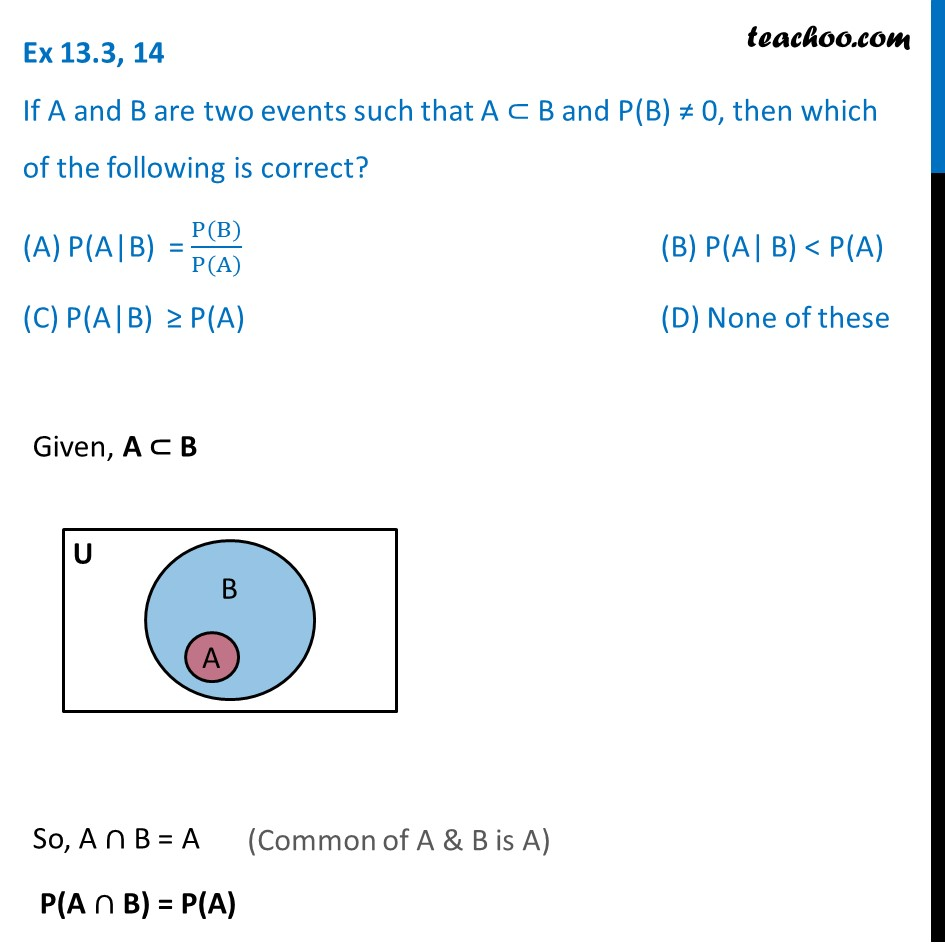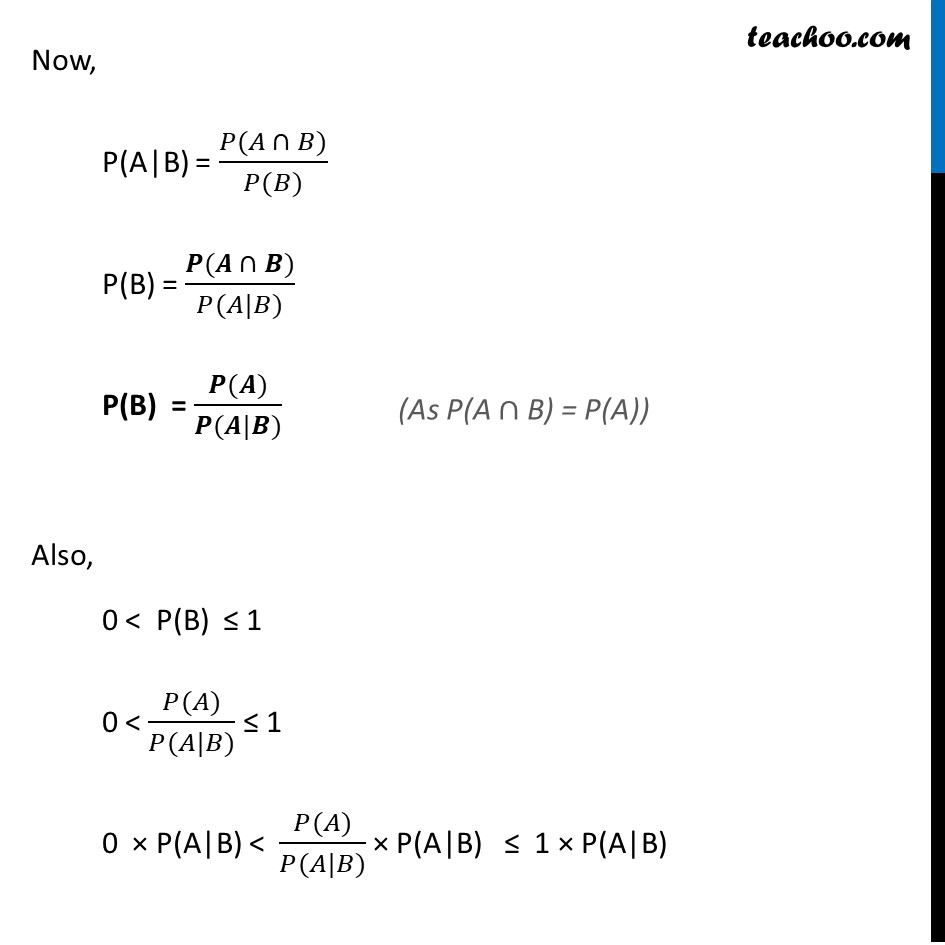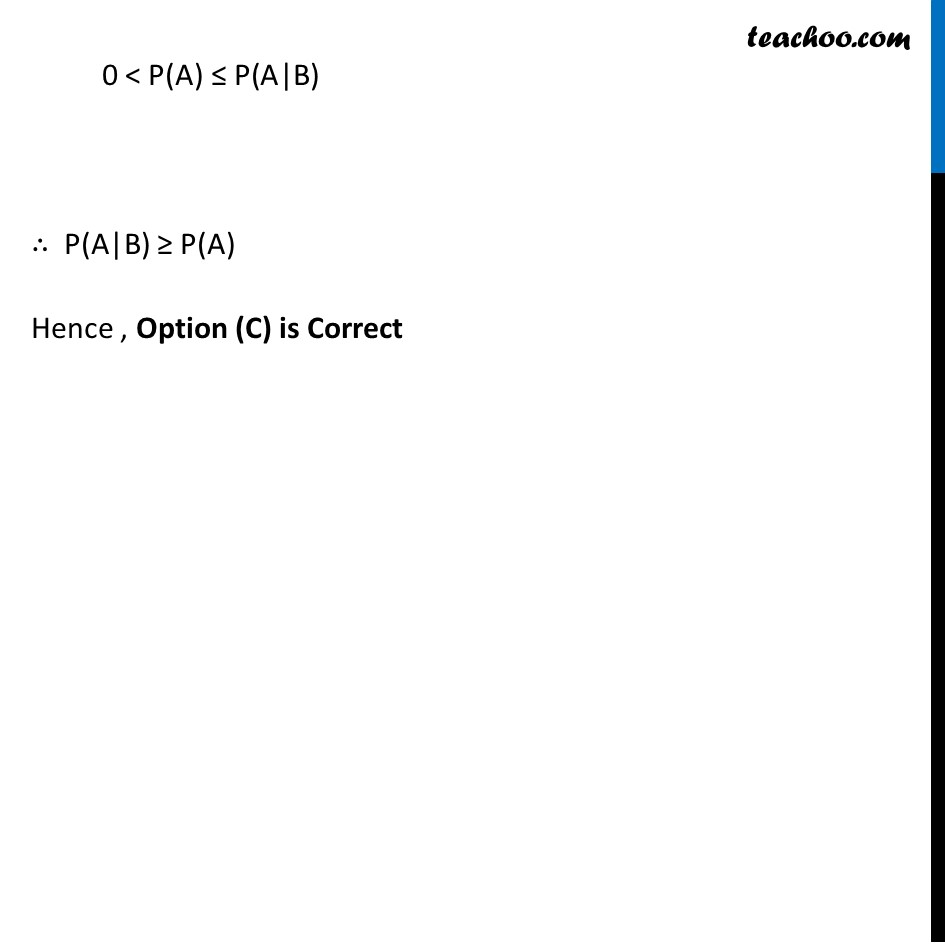Ex 13.3

Chapter 13 Class 12 Probability
Serial order wiseLearn in your speed, with individual attention - Teachoo Maths 1-on-1 Class

### Transcript

Ex 13.3, 14 If A and B are two events such that A ⊂ B and P(B) ≠ 0, then which of the following is correct? (A) P(A|B) = P(B)/P(A) (B) P(A| B) < P(A) (C) P(A|B) ≥ P(A) (D) None of these Given, A ⊂ B So, A ∩ B = A P(A ∩ B) = P(A) Now, P(A|B) = (𝑃(𝐴 ∩ 𝐵))/(𝑃(𝐵)) P(B) = (𝑷(𝑨 ∩ 𝑩))/(𝑃(𝐴|𝐵)) P(B) = (𝑷(𝑨))/(𝑷(𝑨|𝑩)) Also, 0 < "P(B)" ≤ 1 0 < (𝑃(𝐴))/(𝑃(𝐴|𝐵)) ≤ 1 0 × "P(A|B)" < (𝑃(𝐴))/(𝑃(𝐴|𝐵)) × "P(A|B)" ≤ 1 × "P(A|B)" 0 < "P(A) ≤ P(A|B)" ∴ "P(A|B)" ≥ "P(A) " Hence , Option (C) is Correct